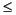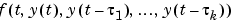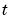MATLAB Function Referenceddeset

Create or alter delay differential equations (DDE) options structure

Syntax

• ```options = ddeset('name1',value1,'name2',value2,...)
options = ddeset(oldopts,'name1',value1,...)
options = ddeset(oldopts,newopts)
ddeset
```

Description

```options = ddeset('name1',value1,'name2',value2,...) ``` creates an integrator options structure `options` in which the named properties have the specified values. Any unspecified properties have default values. It is sufficient to type only the leading characters that uniquely identify the property. `ddeset` ignores case for property names.

```options = ddeset(oldopts,'name1',value1,...) ``` alters an existing options structure `oldopts`. This overwrites any values in `oldopts` that are specified using name/value pairs and returns the modified structure as the output argument.

```options = ddeset(oldopts,newopts) ``` combines an existing options structure `oldopts` with a new options structure `newopts`. Any values set in `newopts` overwrite the corresponding values in `oldopts`.

```ddeset ``` with no input arguments displays all property names and their possible values, indicating defaults with braces `{}`.

You can use the function `ddeget` to query the `options` structure for the value of a specific property.

DDE Properties

The following sections describe the properties that you can set using `ddeset`. There are several categories of properties:

Error Control Properties

At each step, the `dde23` solver estimates the local error `e` in the `i`th component of the solution. This error must be less than or equal to the acceptable error, which is a function of the specified relative tolerance, `RelTol`, and the specified absolute tolerance, `AbsTol`.

• ```|e(i)|max(RelTol*abs(y(i)),AbsTol(i))
```

For routine problems, the `dde23` solver delivers accuracy roughly equivalent to the accuracy you request. It delivers less accuracy for problems integrated over "long" intervals and problems that are moderately unstable. Difficult problems may require tighter tolerances than the default values. For relative accuracy, adjust `RelTol`. For the absolute error tolerance, the scaling of the solution components is important: if `|y|` is somewhat smaller than `AbsTol`, the solver is not constrained to obtain any correct digits in `y`. You might have to solve a problem more than once to discover the scale of solution components.

Roughly speaking, this means that you want `RelTol` correct digits in all solution components except those smaller than thresholds `AbsTol(i)`. Even if you are not interested in a component `y(i)` when it is small, you may have to specify `AbsTol(i)` small enough to get some correct digits in `y(i)` so that you can accurately compute more interesting components

The following table describes the error control properties.

 Property Value Description `RelTol` Positive scalar {`1e-3`} A relative error tolerance that applies to all components of the solution vector `y`. It is a measure of the error relative to the size of each solution component. Roughly, it controls the number of correct digits in all solution components except those smaller than thresholds `AbsTol(i)`. The default, `1e-3`, corresponds to 0.1% accuracy. The estimated error in each integration step satisfies |`e(i)| <= max(RelTol*abs(y(i)),AbsTol(i))`. `AbsTol` Positive scalar or vector {`1e-6`} Absolute error tolerances that apply to the individual components of the solution vector. `AbsTol(i)` is a threshold below which the value of the `i`th solution component is unimportant. The absolute error tolerances determine the accuracy when the solution approaches zero. Even if you are not interested in a component `y(i)` when it is small, you may have to specify `AbsTol(i)` small enough to get some correct digits in `y(i)` so that you can accurately compute more interesting components.If `AbsTol` is a vector, the length of `AbsTol` must be the same as the length of the solution vector `y`. If `AbsTol` is a scalar, the value applies to all components of `y`. `NormControl` `on` | {`off`} Control error relative to norm of solution. Set this property `on` to request that the solvers control the error in each integration step with `norm(e) <= max(RelTol*norm(y),AbsTol)`. By default `dde23` uses a more stringent component-wise error control.

Solver Output Properties

You can use the solver output properties to control the output that the solvers generate.

 Property Value Description `OutputFcn` Function handle {`@odeplot`} The output function is a function that the solver calls after every successful integration step. To specify an output function, set `'OutputFcn'` to a function handle. For example, ```options = ddeset('OutputFcn',@myfun) ``` sets `'OutputFcn'` to `@myfun`, a handle to the function `myfun`. See Function Handles in the MATLAB Programming documentation for more information.The output function must be of the form```status = myfun(t,y,flag) ``` Parameterizing Functions Called by Function Functions, in the MATLAB mathematics documentation, explains how to provide additional parameters to `myfun`, if necessary. The solver calls the specified output function with the following flags. Note that the syntax of the call differs with the flag. The function must respond appropriately:`init` -- The solver calls `myfun(tspan,y0,'init')` before beginning the integration to allow the output function to initialize. `tspan` and `y0` are the input arguments to `dde23`. {none} -- The solver calls `status = myfun(t,y)` after each integration step on which output is requested. `t` contains points where output was generated during the step, and `y` is the numerical solution at the points in `t`. If `t` is a vector, the `i`th column of `y` corresponds to the `i`th element of `t`. `myfun` must return a `status` output value of `0` or `1`. If` status = 1`, the solver halts integration. You can use this mechanism, for instance, to implement a Stop button. done -- The solver calls `myfun([],[],'done') `when integration is complete to allow the output function to perform any cleanup chores. You can use these general purpose output functions or you can edit them to create your own. Type `help functionname` at the command line for more information.`odeplot` - time series plotting (default when you call the solver with no output argument and you have not specified an output function) `odephas2` - two-dimensional phase plane plotting `odephas3` - three-dimensional phase plane plotting `odeprint` - print solution as the solver computes it `OutputSel` Vector of indices Vector of indices specifying which components of the solution vector `dde23` passes to the output function. For example, if you want to use the `ddeplot` output function, but you want to plot only the first and third components of the solution, you can do this using```options = ddeset('OutputFcn',@odeplot,'OutputSel',[1 3]); ``` By default, the solver passes all components of the solution to the output function. `Stats` `on` | {`off`} Specifies whether the solver should display statistics about its computations. By default, `Stats` is `off`. If it is `on`, after solving the problem the solver displays:The number of successful steps The number of failed attempts The number of times the DDE function was called to evaluateStep-Size Properties

The step-size properties let you specify the size of the first step the solver tries, potentially helping it to better recognize the scale of the problem. In addition, you can specify bounds on the sizes of subsequent time steps.

The following table describes the step-size properties.

 Property Value Description `InitialStep` Positive scalar Suggested initial step size. `InitialStep` sets an upper bound on the magnitude of the first step size the solver tries. If you do not set `InitialStep`, the solver bases the initial step size on the slope of the solution at the initial time `tspan(1)`, and the shortest delay. If the slope of all solution components is zero, the procedure might try a step size that is much too large. If you know this is happening or you want to be sure that the solver resolves important behavior at the start of the integration, help the code start by providing a suitable `InitialStep`. `MaxStep ` Positive scalar {`0.1`*`abs(t0-tf)`} Upper bound on solver step size. If the differential equation has periodic coefficients or solutions, it may be a good idea to set `MaxStep` to some fraction (such as 1/4) of the period. This guarantees that the solver does not enlarge the time step too much and step over a period of interest. Do not reduce `MaxStep`:When the solution does not appear to be accurate enough. Instead, reduce the relative error tolerance `RelTol`, and use the solution you just computed to determine appropriate values for the absolute error tolerance vector `AbsTol`. (See Error Control Properties for a description of the error tolerance properties.) To make sure that the solver doesn't step over some behavior that occurs only once during the simulation interval. If you know the time at which the change occurs, break the simulation interval into two pieces and call `dde23` twice. If you do not know the time at which the change occurs, try reducing the error tolerances `RelTol` and `AbsTol`. Use `MaxStep` as a last resort.

Event Location Property

In some DDE problems, the times of specific events are important. While solving a problem, the `dde23` solver can detect such events by locating transitions to, from, or through zeros of user-defined functions.

The following table describes the `Events` property.

 String Value Description `Events` Function handle Handle to a function that includes one or more event functions. See Function Handles in the MATLAB Programming documentation for more information. The function is of the form``` [`value`,`isterminal`,`direction`] = `events`(`t`,y,`Z`) ``` `value`, `isterminal`, and `direction` are vectors for which the `i`th element corresponds to the `i`th event function: `value(i)` is the value of the `i`th event function. `isterminal(i) `= `1` if you want the integration to terminate at a zero of this event function, and `0` otherwise. `direction(i) = 0` if you want `dde23` to locate all zeros (the default), `+1` if only zeros where the event function is increasing, and `-1` if only zeros where the event function is decreasing. If you specify an events function and events are detected, the solver returns three additional fields in the solution structure `sol`:`sol.xe` is a row vector of times at which events occur. `sol.ye` is a matrix whose columns are the solution values corresponding to times in `sol.xe`. `sol.ie` is a vector containing indices that specify which event occurred at the corresponding time in `sol.xe`. For examples that use an event function while solving ordinary differential equation problems, see Example: Simple Event Location (`ballode`) and Example: Advanced Event Location (`orbitode`), in the MATLAB mathematics documentation.

Discontinuity Properties

`dde23` can solve problems with discontinuities in the history or discontinuities in coefficients of the equations. These properties enable you to provide `dde23` with a different initial value, and locations of known discontinuities. See Discontinuities in the MATLAB mathematics documentation for more information.

The following table describes the discontinuity properties.

 String Value Description `Jumps` Vector Location of discontinuities. Pointswhere the history or solution may have a jump discontinuity in a low-order derivative. `InitialY` Vector Initial value of solution. By default the initial value of the solution is the value returned by `history` at the initial point. Supply a different initial value as the value of the `InitialY` property.

Example

To create an options structure that changes the relative error tolerance of the solver from the default value of `1e-3` to `1e-4`, enter

• ```options = ddeset('RelTol', 1e-4);
```

To recover the value of `'RelTol'` from `options`, enter

• ```ddeget(options, 'RelTol')

ans =

1.0000e-004
```

See Also

`dde23`, `ddeget`, `function_handle` (@)

© 1994-2005 The MathWorks, Inc.